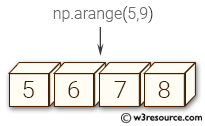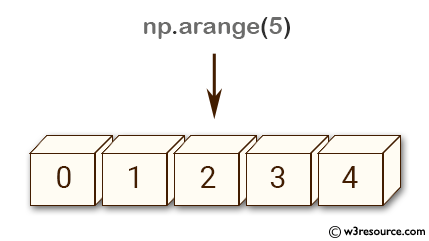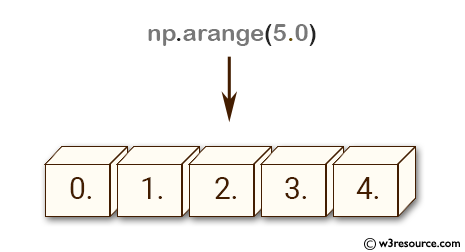# NumPy array creation: arange() function

## numpy.arange() function

The arange() function is used to get evenly spaced values within a given interval.
Values are generated within the half-open interval [start, stop]. For integer arguments the function is equivalent to the Python built-in range function, but returns an ndarray rather than a list.
When using a non-integer step, such as 0.1, the results will often not be consistent. It is better to use linspace for these cases.

Syntax:

`numpy.arange([start, ]stop, [step, ]dtype=None)`Version: 1.15.0

Parameter:

Name Description Required /
Optional
start Start of interval. The interval includes this value. The default start value is 0. Optional
stop End of interval. The interval does not include this value, except in some cases where step is not an integer and floating point round-off affects the length of out. Required
step Spacing between values. For any output out, this is the distance between two adjacent values, out[i+1] - out[i]. The default step size is 1. If step is specified as a position argument, start must also be given. Optional
dtytpe The type of the output array. If dtype is not given, infer the data type from the other input arguments. Optional

Return value:

arange : ndarray - Array of evenly spaced values.
For floating point arguments, the length of the result is ceil((stop - start)/step). Because of floating point overflow, this rule may result in the last element of out being greater than stop.

NumPy.arange() method Example - 1:

``````>>> import numpy as np
>>> np.arange(5)
array([0, 1, 2, 3, 4])
>>> np.arange(5.0)
array([ 0.,  1.,  2.,  3.,  4.])
``````

Pictorial Presentation:NumPy.arange() method Example - 2:

``````>>> import numpy as np
>>> np.arange(5,9)
array([5, 6, 7, 8])
>>> np.arange(5,9,3)
array([5, 8])
``````

Python - NumPy Code Editor: